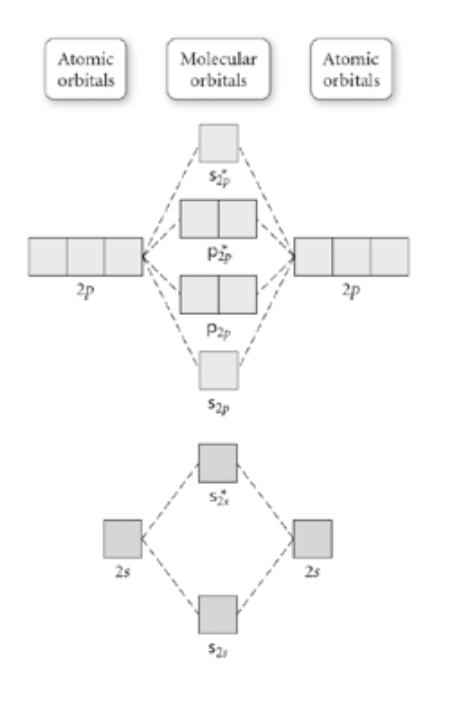# Problem: Use the molecular orbital diagram shown to determine which of the following are paramagnetic.A. Ne22+      B. O22+    C. F22+    D. O22-    E. None of the above are paramagnetic.

###### FREE Expert Solution
86% (360 ratings)
###### Problem Details

Use the molecular orbital diagram shown to determine which of the following are paramagnetic.

A. Ne22+      B. O22+    C. F22+    D. O22-    E. None of the above are paramagnetic.What scientific concept do you need to know in order to solve this problem?

Our tutors have indicated that to solve this problem you will need to apply the Homonuclear Diatomic Molecules concept. If you need more Homonuclear Diatomic Molecules practice, you can also practice Homonuclear Diatomic Molecules practice problems.

What is the difficulty of this problem?

Our tutors rated the difficulty ofUse the molecular orbital diagram shown to determine which o...as medium difficulty.

How long does this problem take to solve?

Our expert Chemistry tutor, Dasha took 6 minutes and 20 seconds to solve this problem. You can follow their steps in the video explanation above.

What professor is this problem relevant for?

Based on our data, we think this problem is relevant for Professor Goldberg's class at VERMONT.